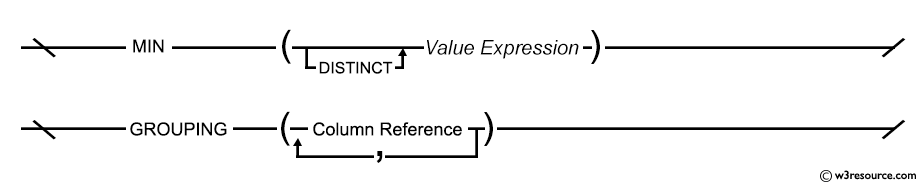# SQL MIN() function

## MIN() function

The aggregate function SQL MIN() is used to find the minimum value or lowest value of a column or expression. This function is useful to determine the smallest of all selected values of a column.

Syntax:

```MIN ([ALL | DISTINCT] expression )
```

## MySQL, PostgreSQL, and SQL Server

All the above platforms support the SQL syntax of MIN.

DB2 and Oracle Syntax:

```MIN ([ALL | DISTINCT] expression ) OVER (window_clause)
```

Parameters:

Name Description
ALL Applies to all values.
DISTINCT Consider each unique value. DISTINCT is not meaningful with MIN function.
expression Expression made up of a single constant, variable, scalar function, or column name or any combination of arithmetic, bitwise, and string operators. MIN can be used with numeric, character, and datetime columns, but not with bit columns. Aggregate functions and subqueries are not permitted.

Syntax diagram - MIN() function## SQL MIN() on specific column

Sample table: orders

To get the minimum or lowest value of 'ord_amout' from the orders table, the following SQL statement can be used:

``````SELECT MIN (ord_amount)
FROM orders;``````

Output:

```MIN(ORD_AMOUNT)
---------------
500
```

## SQL MIN() with addition of two columns

Sample table: customer

To get minimum or lowest values of (opening_amt+receive_amt) from the customer table, the following SQL statement can be used:

```SELECT MIN (opening_amt + receive_amt)
FROM customer;
```

Output:

```MIN(OPENING_AMT+RECEIVE_AMT)
----------------------------
8000
```

## SQL MIN() on date value

Sample table: orders

To get minimum or smallest value of 'ord_date' from the 'orders' table, the following SQL statement can be used:

``````SELECT MIN (ord_date) AS "Min Date"
FROM orders;
``````

Output:

```Min Date
---------
08-JAN-08
```

## All Aggregate FunctionsSee our Model Database

Here is a new document which is a collection of questions with short and simple answers, useful for learning SQL as well as for interviews.

Practice SQL Exercises